×#### Thank you for registering.

One of our academic counsellors will contact you within 1 working day.

Click to Chat

1800-1023-196

+91-120-4616500

CART 0

• 0

MY CART (5)

Use Coupon: CART20 and get 20% off on all online Study Material

ITEM
DETAILS
MRP
DISCOUNT
FINAL PRICE
Total Price: Rs.

There are no items in this cart.
Continue Shopping```Motivation

Table of Content

Meaning of Motivation

Motivation in Mathematical Induction

What is the motivation behind the concept of Mathematical Induction?

Mathematical Deduction

Mathematical Induction

Motivating Mathematical Induction

Principle of Mathematical Induction

When can we use Mathematical Induction?

Meaning of Motivation

Motivation is basically a theoretical concept which is used to describe the behavior of a person or the reaction of a person towards any act. It is a person or a thing which prompts a person to behave in the particular manner. Generally, it is used in psychological theories, to study about the behavior of a person.

Motivation in Mathematical Induction

Motivation in Mathematical Induction means to prove the given statements of n natural numbers in such a way that if it is true for one then it is true for all the other numbers also. It is the method to prove the truthfulness of the entire algorithm.

What is the motivation behind the concept of Mathematical Induction?

It was Euclid who used the concept of decreasing sequence of positive integers which he says should be finite in Book VII, Proposition 1 as a foundation of the theory of numbers of Books VII–IX.Euclid

Then in Book VII, Proposition 31 he used this in more detail that: Any composite number is measured by some prime number.  Check this statement in his proof, "...then an infinite sequence of numbers measures the number A, each of which is less than the other, which is impossible in numbers."Another proof of mathematical induction was there in the al-Fakhri written by al-Karaji which was used by him to prove the properties of Pascal's triangle.

Mathematical Deduction

In mathematics generally we use the concept of deductive reasoning which draws a conclusion from the statement which is already known or assumed.

It comes from the study of logic. If we have given three statements it shows that if the two statements are true then it gives that the third one is also correct.

Examples

Nine is divisible by three.

Any number divisible by nine is divisible by three also, therefore,

Twenty-seven is divisible by three.

It brings the particular conclusion from the general rules.

In mathematics we follow this concept everywhere. If we know the formula of something we always use that formula for solving that particular problem rather than formula is correct or not.

Suppose, it is given that 3x = 9, we will solve this for calculating the value of x by assuming that 3x=9, and we deduce to the conclusion that x = 3.

Mathematical Induction

Mathematical induction is a special method or technique to generalize the statements given in algorithm or to generalize from the particular cases. It is opposite then the deduction method. Here we work on every case and observe each and every case then prove that the given statement is true for all the cases. To prove these statements we use certain principles which are called principle of mathematical induction.

Suppose, we have a set of dominoes and we placed the dominoes in such a way that if we push the first domino then all the dominoes will fall. If we want to be sure for this, we must prove that

The first domino falls

If anyone domino falls then its next one domino or the successor will surely falls.

If both the above statements are proved true then we can say that if we push one domino then all dominoes will fall.Motivating Mathematical Induction

Mathematical Induction gets motivated by the real life examples like if we have a ladder to reach to the roof then how will you provw that you will reach at the top using these ladders.

First thing we need to assure that can we reach to the first step of that ladder, as if we reach to the first step then only you can go to the second, third and so on. And if you will not reach to the first one then you cannot reach to the top.

Second thing is if we count the steps of the ladder then if we assume that we can reach to the 4th step then we can reach to the 5th one also.

Let’s understand it in terms of numbers:

Let’s give the numbers to the ladders as number 1, 2, and so on. Let p (n) is the proposition to reach to the nth number of step. Now we have to prove that if we move to the any step then we can reach to the nth step.

This statement in mathematical term is the same as “for any k ≥ 0, if P (k) is true then P (k + 1) is also true.”

For this first we have to reach to the first step so in mathematical term, If P (1) is true, then only we can say that “if P (k) is true then P (k + 1) is also true” for k = 1 and then we get that P(2) is also true, which then indicates that P(3) is true, and so on, which shows that P(n) is true for all n ≥ 1. But if P (1) is not true, then P (n) also may not be true for any n ≥ 2.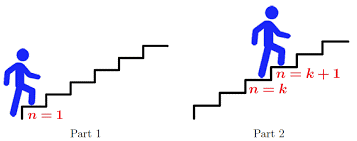Principle of Mathematical Induction

The above concept derives the principle of mathematical induction which is used to directly prove the statements given in terms of n natural numbers.

Base Case: The given statement is correct for first n natural number that is, for n = 1, p (1) is true.

Inductive Step: If the given statement is correct for any natural number like n = k then it will be correct for n = k + 1 also that is, if p (k) is true then p (k+1) is also true.

This principle says that if both the above steps are proven then p (n) is true for all natural numbers.

Example

Let’s take the example of the formula for the sum of the first n squares, for n ≥ 0. We will find a pattern in the sum of squares of n natural numbers:

02 = 0

02 + 12  = 1

02 + 12 + 22 = 5

02 + 12 + 22 + 32 = 14

If we will follow this pattern we can see that the sum of the first n squares could be n (n + 1) (2n + 1)/6. Now we have to prove that this formula works for every number n ≥ 0, that is, we have to prove an infinite number of equalities.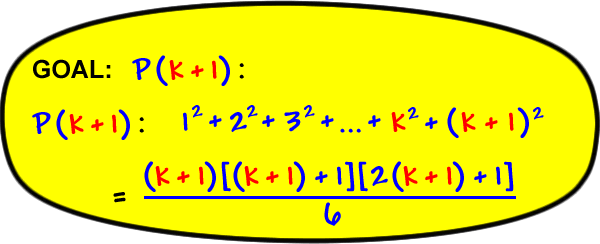Now we will try to prove it with the principle of mathematical induction.

Step 1. First of all we must know that the sum of the first n squares is equal to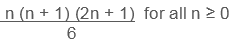Step 2. For any n ≥ 0, let P (n) is the proposition that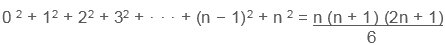We have to show that P (n) is true for all n ≥ 0.

Step 3. In a base case, take n = 0. We will show that P (0) is true: that is,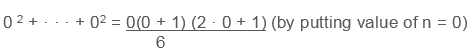02 + · · · + 02 = 0 = 0(0 + 1) (0 + 1)/6

LHS = RHS

For the induction hypothesis, Assume that P (k) is true for  k ≥ 0. that is, assume that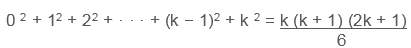Step 4. Now we will prove that P (k + 1) is true by using the above  assumption that P (k) is true. So we will prove thatStep 5. This proves that p (k+1) is true if p (k) is true as follows:

Left-hand side

= 02 + 12 + 22 + · · · + k 2 + (k + 1)2

= (02 + 12 + 22 + · · · + k 2 ) + (k + 1)2Hence, if P (k) is true, then P (k + 1) is also true, for any k ≥ 0.

Step 6. The above steps displayed that for any k ≥ 0, if P(k) is true, then P(k + 1) is also true and  P(0) is also true according to base case, so as both the above two steps are proved then this shows that for all n ≥ 0, P(n) is true, as required.

When can we use Mathematical Induction?

Mathematical induction can be used for so many statements where we want to show, that statement is true. Every statement cannot be proved by this new technique.

First, in induction we need to find our statement P (n) for all integers n ≥ 0, so induction works only for the statements with the whole numbers. So, the statements like “For all x ∈ R, x2 ≥ 0” will not be able to prove with  induction as the set of real numbers is not easy to calculate.

Secondly, for our induction proof we have to follow the inductive step  that is, statements where the P (k+ 1) case is true assuming that the P (k) case is true are particularly well-suited for induction. For Example, statements involving sums  are suitable as it is easy to write the left-hand side of our statement P (k + 1) in terms of the left-hand side of the statement P (k).

Motivation
```### Course Features

• 731 Video Lectures
• Revision Notes
• Previous Year Papers
• Mind Map
• Study Planner
• NCERT Solutions
• Discussion Forum
• Test paper with Video Solution